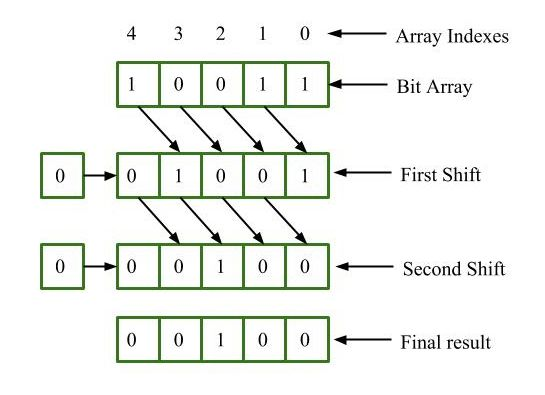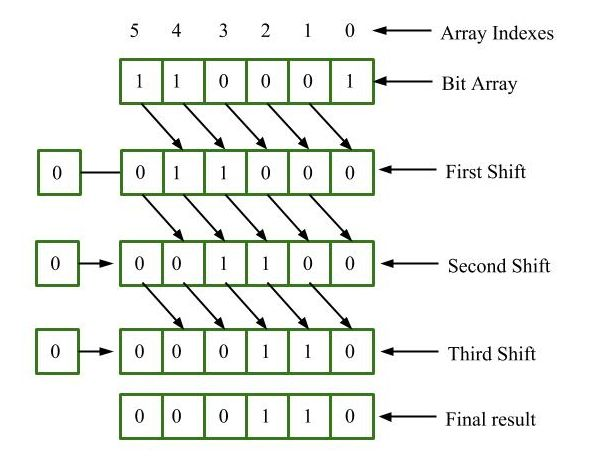GeeksforGeeks App
Open AppBrowser
Continue

# BitArray.RightShift() Method in C# with Examples

BitArray class manages a array of bit values, which are represented as Booleans, where true indicates bit is 1 and false indicates bit is 0. This class is contained in namespace, System.Collections. BitArray.RightShift(Int32) method is used to shift the bits of the bit array to the right by one position and adds zeros on the shifted position. Original BitArray object will be modified on performing the operation right shift.

Syntax: public System.Collections.BitArray RightShift (int count);

Parameter:
count is an immutable value type that represents signed integers with values that range from negative 2,147,483,648 through positive 2,147,483,647.

Return value : It returns Bit Array.

Example 1: Suppose we have the bit array 10011 we want to shift it right by two positions.The final result is 00100.

 `// C# program to illustrate the ``// RightShift(Int32) Method``using` `System;``using` `System.Collections;`` ` `class` `GeeksforGeeks {`` ` `    ``// Main Method``    ``public` `static` `void` `Main()``    ``{`` ` `        ``// Creating a BitArray of ``        ``// size 5 named BitArr``        ``BitArray BitArr = ``new` `BitArray(5);`` ` `        ``// Initializing values in BitArr``        ``BitArr = ``true``;``        ``BitArr = ``true``;``        ``BitArr = ``false``;``        ``BitArr = ``false``;``        ``BitArr = ``true``;`` ` `        ``// function calling``        ``Display(BitArr.RightShift(2));``    ``}`` ` `    ``// Displaying the result``    ``public` `static` `void` `Display(IEnumerable myList)``    ``{``        ``foreach``(Object obj ``in` `myList)``        ``{``            ``Console.WriteLine(obj);``        ``}``    ``}``}`

Output:

```False
False
True
False
False
```

Example 2: Suppose we have the bit array 100011 we want to shift it right by three positions.The final result is 011000.

 `// C# program to illustrate the ``// RightShift(Int32) Method``using` `System;``using` `System.Collections;`` ` `class` `GeeksforGeeks {`` ` `    ``// Main Method``    ``public` `static` `void` `Main()``    ``{`` ` `        ``// Creating a BitArray``        ``BitArray BitArr = ``new` `BitArray(6);`` ` `        ``// Initializing values in BitArr``        ``BitArr = ``true``;``        ``BitArr = ``false``;``        ``BitArr = ``false``;``        ``BitArr = ``false``;``        ``BitArr = ``true``;``        ``BitArr = ``true``;`` ` `        ``// function calling``        ``Display(BitArr.RightShift(3));``    ``}`` ` `    ``// Displaying the result``    ``public` `static` `void` `Display(IEnumerable myList)``    ``{``        ``foreach``(Object obj ``in` `myList)``        ``{``            ``Console.WriteLine(obj);``        ``}``    ``}``}`

Output:

```False
True
True
False
False
False
```

Reference:

My Personal Notes arrow_drop_up